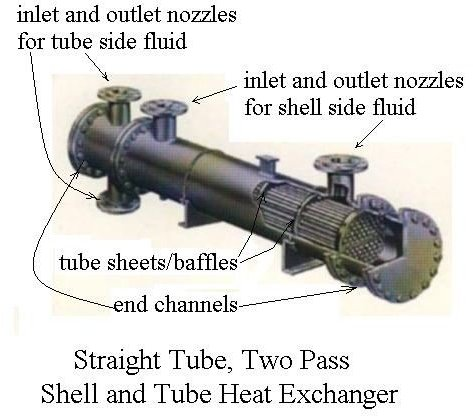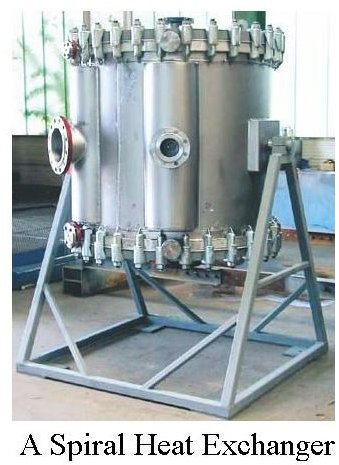# Preliminary Heat Exchanger Design Sizes Heat Exchangers using an Estimated Overall Heat Transfer Coefficient

## Introduction – The Heat Exchanger Design Equation

The basic heat exchanger design equation,

Q = U A ΔTlm,

was introduced and discussed in another article, "Fundamentals of Heat Exchanger Theory and Design." When used as a design equation to calculate the required heat transfer surface area, the equation can be rearranged to become:

A = Q/(U ΔTlm),

where Q is the required heat transfer rate in Btu/hr, U is the overall heat transfer coefficient in Btu/hr-oF, and Δtlm is the log mean temperature difference in oF.

## The Overall Heat Exchanger Design Process

Design of heat exchangers is an iterative (trial & error) process. Here is a set of steps for the process1. Calculate the required heat transfer rate, Q, in Btu/hr from specified information about fluid flow rates and temperatures. (See more details in the next two sections.)
2. Make an initial estimate of the overall heat transfer coefficient, U, based on the fluids involved.
3. Calculate the log mean temperature difference, ΔTlm, from the inlet and outlet temperatures of the two fluids. (See more details in the next two sections.)
4. Calculate the estimated heat transfer area required, using: A = Q/(U ΔTlm).
5. Select a preliminary heat exchanger configuration.
6. Make a more detailed estimate of the overall heat transfer coefficient, U, based on the preliminary heat exchanger configuration.
7. Estimate the pressure drop across the heat exchanger. If it is too high, revise the heat exhcanger configuration until the pressure drop is acceptable.
8. If the new estimate of U is significantly different than the previous estimate, repeat steps 4 through 7 as many times as necessary until the two estimates are the same to the desired degree of accuracy.

## Input Information Needed

In order to start the heat exchanger design process, several items of information are needed as follows:

1. The two fluids involved need to be identified.
2. The heat capacity of each fluid is needed.
3. The required initial and final temperatures for one of the fluids are needed.
4. The design value of the initial temperature for the other fluid is needed.
5. An initial estimate for the value of the Overall Heat Transfer Coefficient, U, is needed.Knowing the first four items allows determination of the required heat transfer rate, Q, and the inlet and outlet temperatures of both fluids, thus allowing calculation of the log mean temperature difference, ΔTlm. With values now available for Q, U, and ΔTlm, an initial estimate for the required heat transfer area can be calculated from the equation,

A = Q/(U ΔTlm).

## Example Calculations

Example #1: Calculate a preliminary estimate of the heat exchanger area needed to cool 55,000 lb/hr of a light oil (specific heat = 0.74 Btu/lb-oF) from 190oF to 140oF using cooling water that is available at 50oF. The cooling water can be allowed to heat to 90oF. An initial estimate of the overall heat transfer coefficient is 120 Btu/hr-ft2oF. Also estimate the required mass flow rate of cooling water.

Solution: First calculate the required heat transfer rate based on the required light oil cooling:

Q = (55,000 lb/hr)(0.74 Btu/lb-oF)(190 – 140)oF = 2,035,000 Btu/hr.

Next calculate the log mean temperature difference:

ΔTlm = [(190 – 90) – (140 – 50)]/ln[(190 – 90)/(140 – 50)] = 94.9oF

The preliminary area estimate can now be calculated as:

A = Q/(U ΔTlm) = 2,035,000/(120)(79.58) = 178.7 ft2 = A

The required mass flow rate of water can be calculated from Q = m Cp ΔT.

Rearranging: m = Q/Cp ΔT = (2,035,000 Btu/hr)/(1 Btu/lb-oF)(40oF) = 50,875 lb/hr

Example #2: A shell and tube heat exchanger is to be used for the light oil cooling described in Example #1. How many tubes of 3 inch diameter and 10 ft length should be used?

Solution: The surface area per tube will be πDL = π(3/12)(10) ft2 = 7.854 ft2. The number of tubes required would thus be:

n = 178.7 ft2/7.854 ft2/tube = 22.7 tubes (round up to 23 tubes).

The next step would be to check on the pressure drop for this tube configuration and the specified flow. If the pressure drop is acceptable, then the overall heat transfer coefficient could be re-estimated for this heat exchanger configuration. These topics will be dealt with in a future article.

## Summary

Calculations for preliminary design of heat exchangers are illustrated through the examples in this article. The basic heat exchanger design equation is the centerpiece of the process and uses the overall heat transfer coefficient and log mean temperature difference in the calculations.

For downloadable Excel spreadsheet templates to make preliminary shell and tube and double pipe heat exchanger design calculations, see the article, "Excel Spreadsheet Templates for Preliminary Heat Exchanger Design Calculations."

## References and Image Credits

References for Further Information:

1.Bengtson, H., Fundamentals of Heat Exchangers, an online, continuing education course for PDH credit.

2. Bengtson, H., Excel Spreadsheets for Preliminary Heat Exchanger Design.

2. Kuppan, T., Heat Exchanger Design Handbook, CRC Press, 2000.

3. Kakac, S. and Liu, H., Heat Exchangers: Selection, Rating and Thermal Design, CRC Press, 2002.

Image Credits:

Shell and Tube Heat Exchanger – Grand Kartech heat exchangers

A Spiral Heat Exchanger Picture – Tranter/Europe

## This post is part of the series: Heat Exchanger Design

Heat exchanger design includes estimation of the heat transfer area needed for known or estimated heat transfer rate, overall heat transfer coefficient and log mean temperature difference. The tube or pipe diameters and length also need to be determined, as well as the pressure drop.